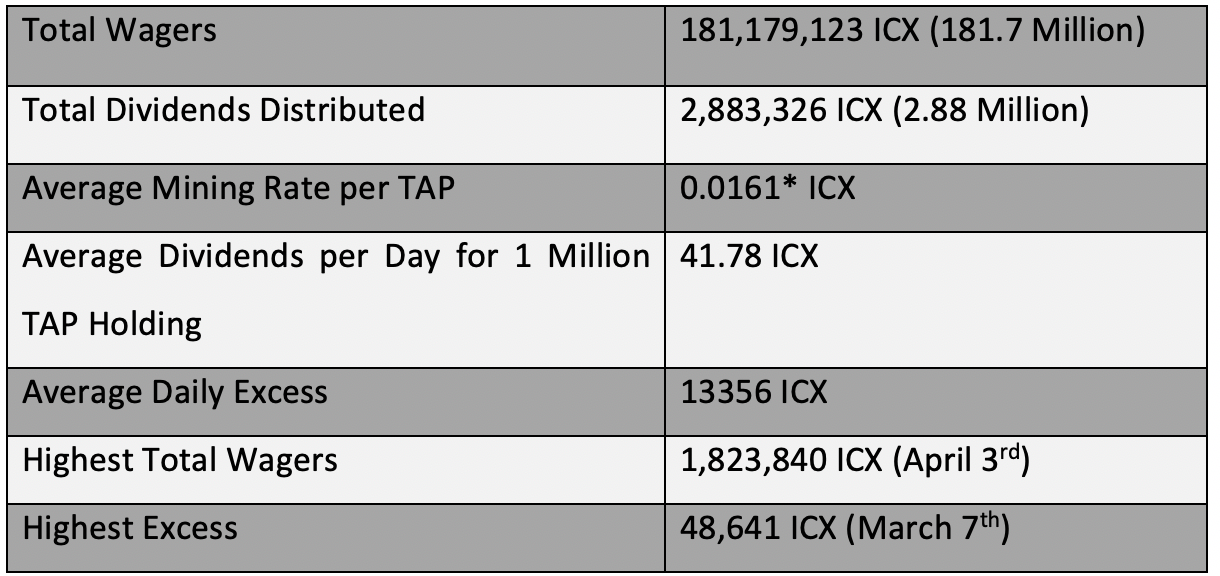# ICONbet Statistics for the period January 1st,2020 — July 31st, 2020

The below statistics have been compiled using the publicly available data and the data shared by the ICONbet community member.*Average mining rate is calculated based on just the excess, if the developer fee is to be considered the average mining rate would be up to 20% more.

If you had 1 Million TAP by Dec 31st, 2019, you would have made 8899 ICX in the following 7 Months.

Following would be your ROI based on your cost of acquiring TAP.

If cost of 1 Million TAP = 12000 ICX.
ROI for 7 Months would be =(8899/12000)*100 =74.15%

If cost of 1 Million TAP = 14000 ICX.
ROI for 7 Months would be =(8899/14000)*100 =63.56%

If cost of 1 Million TAP = 16000 ICX.
ROI for 7 Months would be =(8899/16000)*100 =55.61%

If cost of 1 Million TAP = 18000 ICX.
ROI for 7 Months would be=(8899/18000)*100 =49.43%

If cost of 1 Million TAP = 20000 ICX.
ROI for 7 Months would be =(8899/20000)*100 =44.49%

If cost of 1 Million TAP = 22000 ICX.
ROI for 7 Months would be =(8899/22000)*100 =40.45%

If cost of 1 Million TAP = 24000 ICX.
ROI for 7 Months would be=(8899/24000)*100 =37.70%

If cost of 1 Million TAP = 26000 ICX.
ROI for 7 Months would be=(8899/26000)*100 =34.22%

If cost of 1 Million TAP = 28000 ICX.
ROI for 7 Months would be=(8899/28000)*100 =31.78%

If cost of 1 Million TAP = 30000 ICX.
ROI for 7 Months would be=(8899/30000)*100 =29.66%

Note: If you like what we are doing, please vote for ICONbet Community P-Rep Node. Every vote and every voter counts. Also all members of the ICONbet Community P-Rep team are volunteers.

https://iconbet.io/